Guru

# Form the pair of linear equations in the following problems, and find their solutions graphically. (i) 10 students of Class X took part in a Mathematics quiz. If the number of girls is 4 more than the number of boys, find the number of boys and girls who took part in the quiz. (ii) 5 pencils and 7 pens together cost 50, whereas 7 pencils and 5 pens together cost 46. Find the cost of one pencil and that of one pen. Q.1

• 0

How can i solve this lengthy question of class 10th math of exercise 3.2. How i solve easily this question. I have tried many times for solve this question but it’s not. so please help me for solving this question Form the pair of linear equations in the following problems, and find their solutions graphically. (i) 10 students of Class X took part in a Mathematics quiz. If the number of girls is 4 more than the number of boys, find the number of boys and girls who took part in the quiz. (ii) 5 pencils and 7 pens together cost 50, whereas 7 pencils and 5 pens together cost 46. Find the cost of one pencil and that of one pen.

Share

1. (i)Let there are x number of girls and y number of boys. As per the given question, the algebraic expression can be represented as follows.

x +y = 10

x– y = 4

Now, for x+y = 10 or x = 10−y, the solutions are;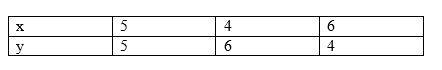For x – y = 4 or x = 4 + y, the solutions are;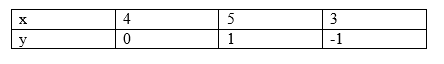The graphical representation is as follows;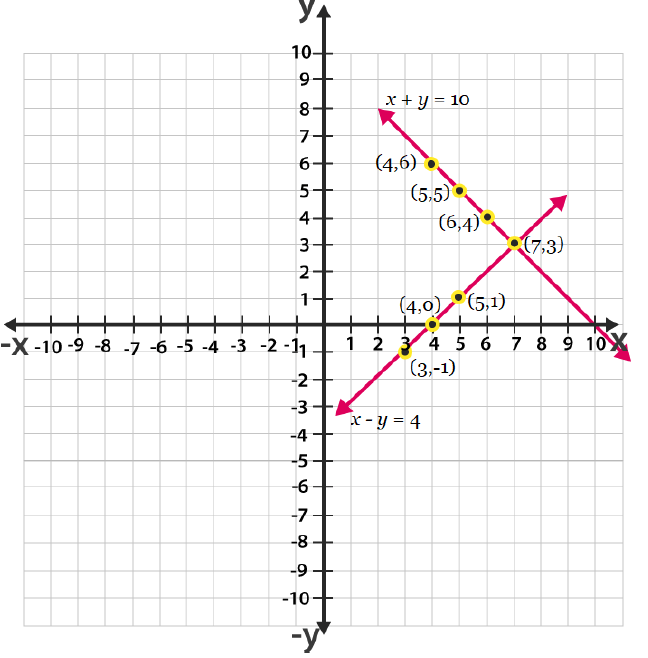From the graph, it can be seen that the given lines cross each other at point (7, 3). Therefore, there are 7 girls and 3 boys in the class.

(ii) Let 1 pencil costs Rs.x and 1 pen costs Rs.y.

According to the question, the algebraic expression cab be represented as;

5x + 7y = 50

7x + 5y = 46

For, 5x + 7y = 50 or  x = (50-7y)/5, the solutions are;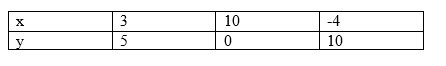For 7x + 5y = 46 or x = (46-5y)/7, the solutions are;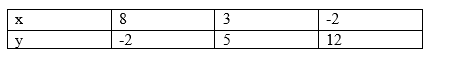Hence, the graphical representation is as follows;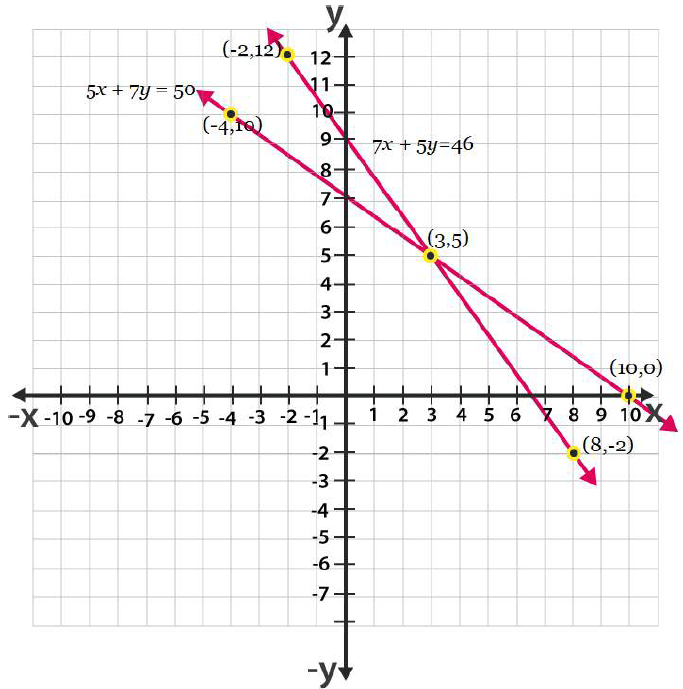From the graph, it is can be seen that the given lines cross each other at point (3, 5).

So, the cost of a pencil is 3/- and cost of a pen is 5/-.

• 0